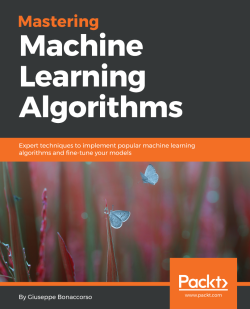# Mastering Machine Learning Algorithms4.4 (11 reviews total)
By Giuseppe Bonaccorso
• FREE Subscription Access now
• \$10.00 Was 35.99 eBook
What do you get with a Packt Subscription?

• Constantly updated with 100+ new titles each month
• Breadth and depth in over 1,000+ technologies

Machine learning is a subset of AI that aims to make modern-day computer systems smarter and more intelligent. The real power of machine learning resides in its algorithms, which make even the most difficult things capable of being handled by machines. However, with the advancement in the technology and requirements of data, machines will have to be smarter than they are today to meet the overwhelming data needs; mastering these algorithms and using them optimally is the need of the hour.

Mastering Machine Learning Algorithms is your complete guide to quickly getting to grips with popular machine learning algorithms. You will be introduced to the most widely used algorithms in supervised, unsupervised, and semi-supervised machine learning, and will learn how to use them in the best possible manner. Ranging from Bayesian models to the MCMC algorithm to Hidden Markov models, this book will teach you how to extract features from your dataset and perform dimensionality reduction by making use of Python-based libraries such as scikit-learn. You will also learn how to use Keras and TensorFlow to train effective neural networks.

If you are looking for a single resource to study, implement, and solve end-to-end machine learning problems and use-cases, this is the book you need.

Publication date:
May 2018
Publisher
Packt
Pages
576
ISBN
9781788621113

## Chapter 1. Machine Learning Model Fundamentals

Machine learning models are mathematical systems that share many common features. Even if, sometimes, they have been defined only from a theoretical viewpoint, research advancement allows us to apply several concepts to better understand the behavior of complex systems such as deep neural networks. In this chapter, we're going to introduce and discuss some fundamental elements that some skilled readers may already know, but that, at the same time, offer several possible interpretations and applications.

In particular, in this chapter we're discussing the main elements of:

• Data-generating processes
• Finite datasets
• Training and test split strategies
• Cross-validation
• Capacity, bias, and variance of a model
• Vapnik-Chervonenkis theory
• Cramér-Rao bound
• Underfitting and overfitting
• Loss and cost functions
• Regularization

## Models and data

Machine learning algorithms work with data. They create associations, find out relationships, discover patterns, generate new samples, and more, working with well-defined datasets. Unfortunately, sometimes the assumptions or the conditions imposed on them are not clear, and a lengthy training process can result in a complete validation failure. Even if this condition is stronger in deep learning contexts, we can think of a model as a gray box (some transparency is guaranteed by the simplicity of many common algorithms), where a vectorial inputis transformed into a vectorial output: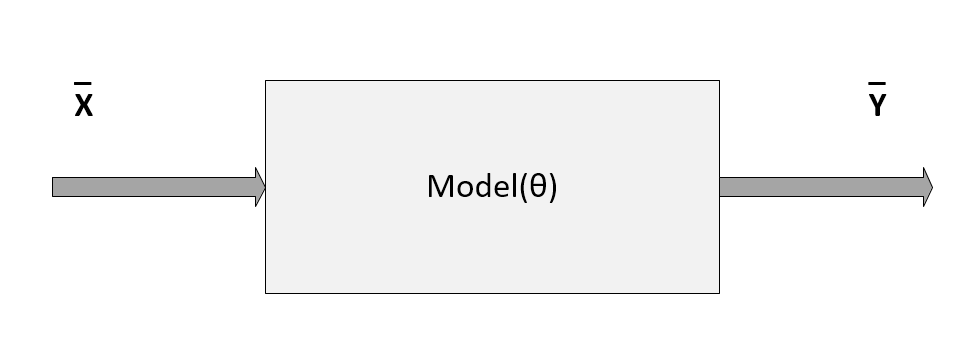Schema of a generic model parameterized with the vector θ

In the previous diagram, the model has been represented by a pseudo-function that depends on a set of parameters defined by the vector θ. In this section, we are only considering parametric models, although there's a family of algorithms that are called non-parametric, because they are based only on the structure of the data. We're going to discuss some of them in upcoming chapters.

The task of a parametric learning process is therefore to find the best parameter set that maximizes a target function whose value is proportional to the accuracy (or the error, if we are trying to minimize them) of the model given a specific input X and output Y. This definition is not very rigorous, and it will be improved in the following sections; however, it's useful as a way to understand the context we're working in.

Then, the first question to ask is: What is the nature of X? A machine learning problem is focused on learning abstract relationships that allow a consistent generalization when new samples are provided. More specifically, we can define a stochastic data generating process with an associated joint probability distribution:Sometimes, it's useful to express the joint probability p(x, y) as a product of the conditional p(y|x), which expresses the probability of a label given a sample, and the marginal probability of the samples p(x). This expression is particularly useful when the prior probability p(x) is known in semi-supervised contexts, or when we are interested in solving problems using the Expectation Maximization (EM) algorithm. We're going to discuss this approach in upcoming chapters.

In many cases, we are not able to derive a precise distribution; however, when considering a dataset, we always assume that it's drawn from the original data-generating distribution. This condition isn't a purely theoretical assumption, because, as we're going to see, whenever our data points are drawn from different distributions, the accuracy of the model can dramatically decrease.

If we sample N independent and identically distributed (i.i.d.) values from pdata, we can create a finite dataset X made up of k-dimensional real vectors:In a supervised scenario, we also need the corresponding labels (with t output values):When the output has more than two classes, there are different possible strategies to manage the problem. In classical machine learning, one of the most common approaches is One-vs-All, which is based on training N different binary classifiers where each label is evaluated against all the remaining ones. In this way, N-1 is performed to determine the right class. With shallow and deep neural networks, instead, it's preferable to use a softmax function to represent the output probability distribution for all classes:This kind of output (zi represents the intermediate values, and the sum of the terms is normalized to 1) can be easily managed using the cross-entropy cost function (see the corresponding paragraph in the Loss and cost functions section).

### Zero-centering and whitening

Many algorithms show better performances (above all, in terms of training speed) when the dataset is symmetric (with a zero-mean). Therefore, one of the most important preprocessing steps is so-called zero-centering, which consists in subtracting the feature-wise mean Ex[X] from all samples:This operation, if necessary, is normally reversible, and doesn't alter relationships both among samples and among components of the same sample. In deep learning scenarios, a zero-centered dataset allows exploiting the symmetry of some activation function, driving to a faster convergence (we're going to discuss these details in the next chapters).

Another very important preprocessing step is called whitening, which is the operation of imposing an identity covariance matrix to a zero-centered dataset:As the covariance matrix Ex[XTX] is real and symmetric, it's possible to eigendecompose it without the need to invert the eigenvector matrix:The matrix V contains the eigenvectors (as columns), and the diagonal matrix Ω contains the eigenvalues. To solve the problem, we need to find a matrix A, such that:Using the eigendecomposition previously computed, we get:Hence, the matrix A is:One of the main advantages of whitening is the decorrelation of the dataset, which allows an easier separation of the components. Furthermore, if X is whitened, any orthogonal transformation induced by the matrix P is also whitened:Moreover, many algorithms that need to estimate parameters that are strictly related to the input covariance matrix can benefit from this condition, because it reduces the actual number of independent variables (in general, these algorithms work with matrices that become symmetric after applying the whitening). Another important advantage in the field of deep learning is that the gradients are often higher around the origin, and decrease in those areas where the activation functions (for example, the hyperbolic tangent or the sigmoid) saturate (|x| → ∞). That's why the convergence is generally faster for whitened (and zero-centered) datasets.

In the following graph, it's possible to compare an original dataset,zero-centering, and whitening: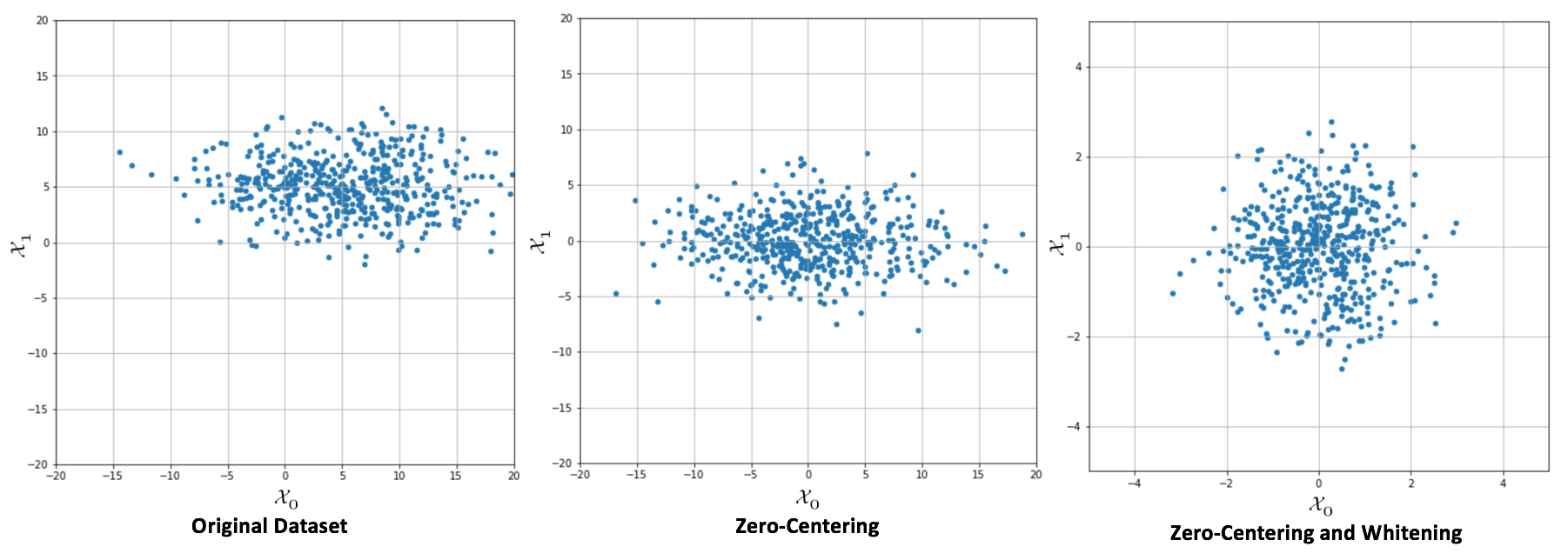Original dataset (left), centered version (center), whitened version (right)

When a whitening is needed, it's important to consider some important details. The first one is that there's a scale difference between the real sample covariance and the estimation XTX, often adopted with the singular value decomposition (SVD). The second one concerns some common classes implemented by many frameworks, like Scikit-Learn's `StandardScaler`. In fact, while zero-centering is a feature-wise operation, a whitening filter needs to be computed considering the whole covariance matrix (`StandardScaler` implements only unit variance, feature-wise scaling).

Luckily, all Scikit-Learn algorithms that benefit from or need a whitening preprocessing step provide a built-in feature, so no further actions are normally required; however, for all readers who want to implement some algorithms directly, I've written two Python functions that can be used both for zero-centering and whitening. They assume a matrix X with a shape (NSamples × n). Moreover, the `whiten()` function accepts the parameter `correct`, which allows us to apply the scaling correction (the default value is `True`):

```import numpy as np

def zero_center(X):
return X - np.mean(X, axis=0)

def whiten(X, correct=True):
Xc = zero_center(X)
_, L, V = np.linalg.svd(Xc)
W = np.dot(V.T, np.diag(1.0 / L))
return np.dot(Xc, W) * np.sqrt(X.shape) if correct else 1.0```

### Training and validation sets

In real problems, the number of samples is limited, and it's usually necessary to split the initial set X (together with Y) into two subsets as follows:

• Training set used to train the model
• Validation set used to assess the score of the model without any bias, with samples never seen before

According to the nature of the problem, it's possible to choose a split percentage ratio of 70% – 30% (a good practice in machine learning, where the datasets are relatively small), or a higher training percentage (80%, 90%, up to 99%) for deep learning tasks where the number of samples is very high. In both cases, we are assuming that the training set contains all the information required for a consistent generalization. In many simple cases, this is true and can be easily verified; but with more complex datasets, the problem becomes harder. Even if we think to draw all the samples from the same distribution, it can happen that a randomly selected test set contains features that are not present in other training samples. Such a condition can have a very negative impact on global accuracy and, without other methods, it can also be very difficult to identify. This is one of the reasons why, in deep learning, training sets are huge: considering the complexity of the features and structure of the data generating distributions, choosing large test sets can limit the possibility of learning particular associations.

In Scikit-Learn, it's possible to split the original dataset using the `train_test_split()` function, which allows specifying the train/test size, and if we expect to have randomly shuffled sets (default). For example, if we want to split `X` and `Y`, with 70% training and 30% test, we can use:

```from sklearn.model_selection import train_test_split

X_train, X_test, Y_train, Y_test = train_test_split(X, Y, train_size=0.7, random_state=1)```

Shuffling the sets is always a good practice, in order to reduce the correlation between samples. In fact, we have assumed that `X` is made up of i.i.d samples, but several times two subsequent samples have a strong correlation, reducing the training performance. In some cases, it's also useful to re-shuffle the training set after each training epoch; however, in the majority of our examples, we are going to work with the same shuffled dataset throughout the whole process. Shuffling has to be avoided when working with sequences and models with memory: in all those cases, we need to exploit the existing correlation to determine how the future samples are distributed.

### Note

When working with NumPy and Scikit-Learn, it's always a good practice to set the random seed to a constant value, so as to allow other people to reproduce the experiment with the same initial conditions. This can be achieved by calling `np.random.seed`(...) and using the `random-state` parameter present in many Scikit-Learn methods.

#### Cross-validation

A valid method to detect the problem of wrongly selected test sets is provided by the cross-validation technique. In particular, we're going to use the K-Fold cross-validation approach. The idea is to split the whole dataset X into a moving test set and a training set (the remaining part). The size of the test set is determined by the number of folds so that, during k iterations, the test set covers the whole original dataset.

In the following diagram, we see a schematic representation of the process: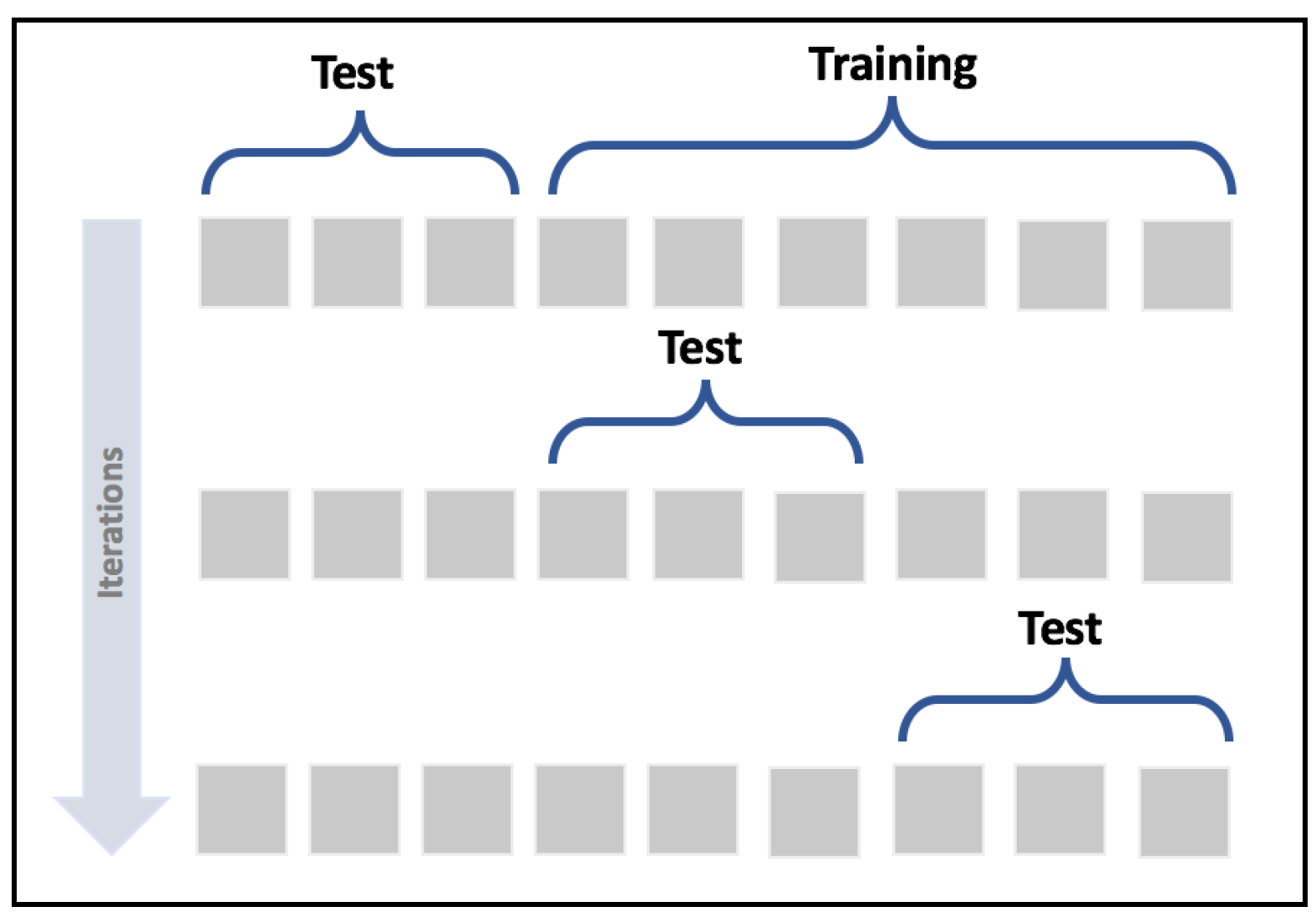K-Fold cross-validation schema

In this way, it's possible to assess the accuracy of the model using different sampling splits, and the training process can be performed on larger datasets; in particular, on (k-1)*N samples. In an ideal scenario, the accuracy should be very similar in all iterations; but in most real cases, the accuracy is quite below average. This means that the training set has been built excluding samples that contain features necessary to let the model fit the separating hypersurface considering the real pdata. We're going to discuss these problems later in this chapter; however, if the standard deviation of the accuracies is too high (a threshold must be set according to the nature of the problem/model), that probably means that X hasn't been drawn uniformly from pdata, and it's useful to evaluate the impact of the outliers in a preprocessing stage. In the following graph, we see the plot of a 15-fold cross-validation performed on a logistic regression: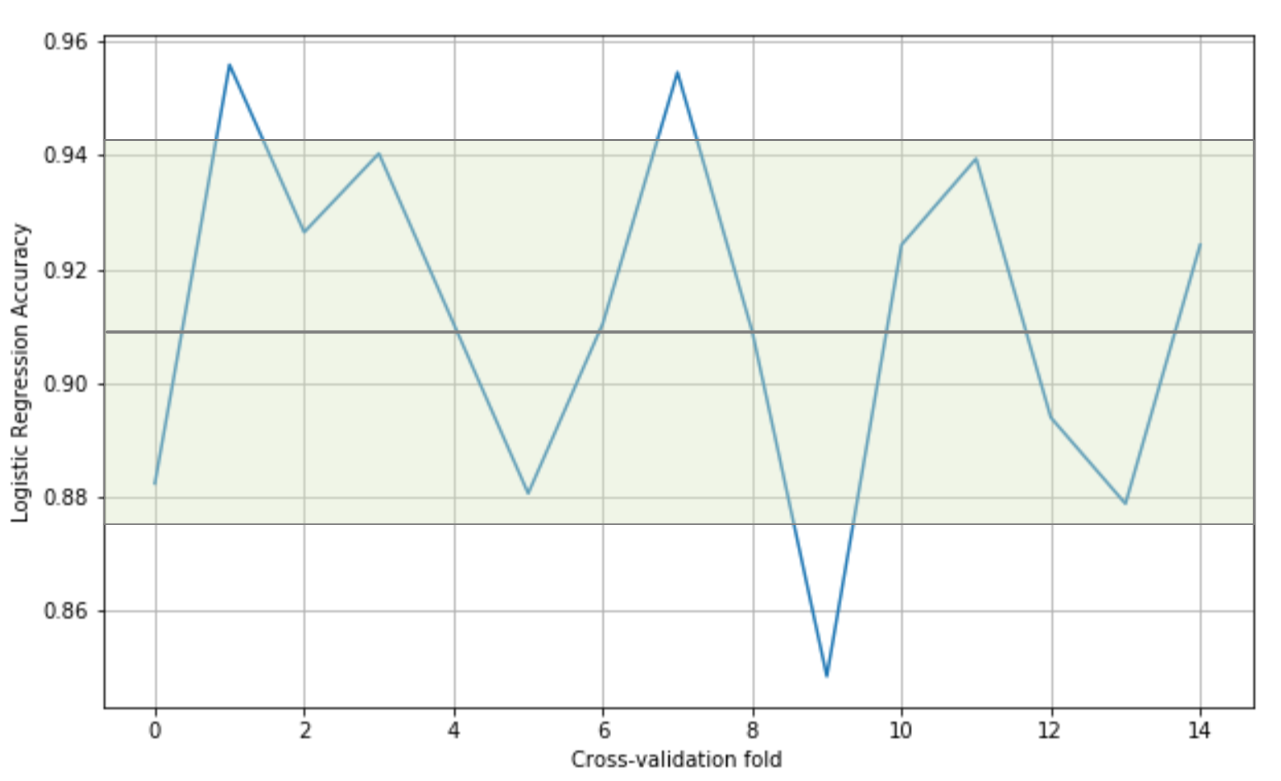Cross-validation accuracies

The values oscillate from 0.84 to 0.95, with an average (solid horizontal line) of 0.91. In this particular case, considering the initial purpose was to use a linear classifier, we can say that all folds yield high accuracies, confirming that the dataset is linearly separable; however, there are some samples (excluded in the ninth fold) that are necessary to achieve a minimum accuracy of about 0.88.

K-Fold cross-validation has different variants that can be employed to solve specific problems:

• Stratified K-Fold: A Standard K-Fold approach splits the dataset without considering the probability distribution p(y|x), therefore some folds may theoretically contain only a limited number of labels. Stratified K-Fold, instead, tries to split X so that all the labels are equally represented.
• Leave-one-out (LOO): This approach is the most drastic because it creates N folds, each of them containing N-1 training samples and only 1 test sample. In this way, the maximum possible number of samples is used for training, and it's quite easy to detect whether the algorithm is able to learn with sufficient accuracy, or if it's better to adopt another strategy. The main drawback of this method is that N models must be trained, and when N is very large this can cause a performance issue. Moreover, with a large number of samples, the probability that two random values are similar increases, therefore many folds will yield almost identical results. At the same time, LOO limits the possibilities for assessing the generalization ability, because a single test sample is not enough for a reasonable estimation.
• Leave-P-out (LPO): In this case, the number of test samples is set to p (non-disjoint sets), so the number of folds is equal to the binomial coefficient of n over p. This approach mitigates LOO's drawbacks, and it's a trade-off between K-Fold and LOO. The number of folds can be very high, but it's possible to control it by adjusting the number p of test samples; however, if p isn't small or big enough, the binomial coefficient can explode. In fact, when p has about n/2 samples, the number of folds is maximal:Scikit-Learn implements all those methods (with some other variations), but I suggest always using the `cross_val_score()` function, which is a helper that allows applying the different methods to a specific problem. In the following snippet based on a polynomial Support Vector Machine (SVM) and the MNIST digits dataset, the function is applied specifying the number of folds (parameter `cv`). In this way, Scikit-Learn will automatically use Stratified K-Fold for categorical classifications, and Standard K-Fold for all other cases:

```from sklearn.datasets import load_digits
from sklearn.model_selection import cross_val_score
from sklearn.svm import SVC

svm = SVC(kernel='poly')

skf_scores = cross_val_score(svm, data['data'], data['target'], cv=10)

print(skf_scores)
[ 0.96216216  1.          0.93922652  0.99444444  0.98882682  0.98882682
0.99441341  0.99438202  0.96045198  0.96590909]

print(skf_scores.mean())
0.978864325583
```

The accuracy is very high (> 0.9) in every fold, therefore we expect to have even higher accuracy using the LOO method. As we have 1,797 samples, we expect the same number of accuracies:

```from sklearn.model_selection import cross_val_score, LeaveOneOut

loo_scores = cross_val_score(svm, data['data'], data['target'], cv=LeaveOneOut())

print(loo_scores[0:100])
[ 1.  1.  1.  1.  1.  0.  1.  1.  1.  1.  1.  1.  1.  1.  1.  1.  1.  1.
1.  1.  1.  1.  1.  1.  1.  1.  1.  1.  1.  1.  1.  1.  1.  1.  1.  1.
1.  0.  1.  1.  1.  1.  1.  1.  1.  1.  1.  1.  1.  1.  1.  1.  1.  1.
1.  1.  1.  1.  1.  1.  1.  1.  1.  1.  1.  1.  1.  1.  1.  0.  1.  1.
1.  1.  1.  1.  1.  1.  1.  1.  1.  1.  1.  1.  1.  1.  1.  1.  1.  1.
1.  1.  1.  1.  1.  1.  1.  1.  1.  1.]

print(loo_scores.mean())
0.988870339455```

As expected, the average score is very high, but there are still samples that are misclassified. As we're going to discuss, this situation could be a potential candidate for overfitting, meaning that the model is learning perfectly how to map the training set, but it's losing its ability to generalize; however, LOO is not a good method to measure this model ability, due to the size of the validation set.

We can now evaluate our algorithm with the LPO technique. Considering what was explained before, we have selected the smaller Iris dataset and a classification based on a logistic regression. As there are N=150 samples, choosing `p = 3`, we get 551,300 folds:

```from sklearn.datasets import load_iris
from sklearn.linear_model import LogisticRegression
from sklearn.model_selection import cross_val_score, LeavePOut

p = 3
lr = LogisticRegression()

lpo_scores = cross_val_score(lr, data['data'], data['target'], cv=LeavePOut(p))

print(lpo_scores[0:100])
[ 1.          1.          1.          1.          1.          1.          1.
1.          1.          1.          1.          1.          1.          1.
1.          1.          1.          1.          1.          1.          1.
1.          1.          1.          1.          1.          1.          1.
1.          1.          1.          1.          1.          1.          1.
1.          1.          1.          1.          1.          1.          1.
1.          1.          1.          1.          1.          1.          1.
1.          1.          1.          1.          1.          1.          1.
1.          1.          1.          1.          1.          1.          1.
1.          0.66666667  ...

print(lpo_scores.mean())
0.955668420098```

As in the previous example, we have printed only the first 100 accuracies; however, the global trend can be immediately understood with only a few values.

The cross-validation technique is a powerful tool that is particularly useful when the performance cost is not too high. Unfortunately, it's not the best choice for deep learning models, where the datasets are very large and the training processes can take even days to complete. However, as we're going to discuss, in those cases the right choice (the split percentage), together with an accurate analysis of the datasets and the employment of techniques such as normalization and regularization, allows fitting models that show an excellent generalization ability.

## Features of a machine learning model

In this section, we're going to consider supervised models, and try to determine how it's possible to measure their theoretical potential accuracy and their ability to generalize correctly over all possible samples drawn from pdata. The majority of these concepts were developed before the deep learning age, but continue to have an enormous influence on research projects. The idea of capacity, for example, is an open-ended question that neuroscientists keep on asking themselves about the human brain. Modern deep learning models with dozens of layers and millions of parameters reopened the theoretical question from a mathematical viewpoint. Together with this, other elements, like the limits for the variance of an estimator, again attracted the limelight because the algorithms are becoming more and more powerful, and performances that once were considered far from any feasible solution are now a reality. Being able to train a model, so as to exploit its full capacity, maximize its generalization ability, and increase the accuracy, overcoming even human performances, is what a deep learning engineer nowadays has to expect from his work.

### Capacity of a model

If we consider a supervised model as a set of parameterized functions, we can define representational capacity as the intrinsic ability of a certain generic function to map a relatively large number of data distributions. To understand this concept, let's consider a function f(x) that admits infinite derivatives, and rewrite it as a Taylor expansion:We can decide to take only the first n terms, so to have an n-degree polynomial function. Consider a simple bi-dimensional scenario with six functions (starting from a linear one); we can observe the different behavior with a small set of data points: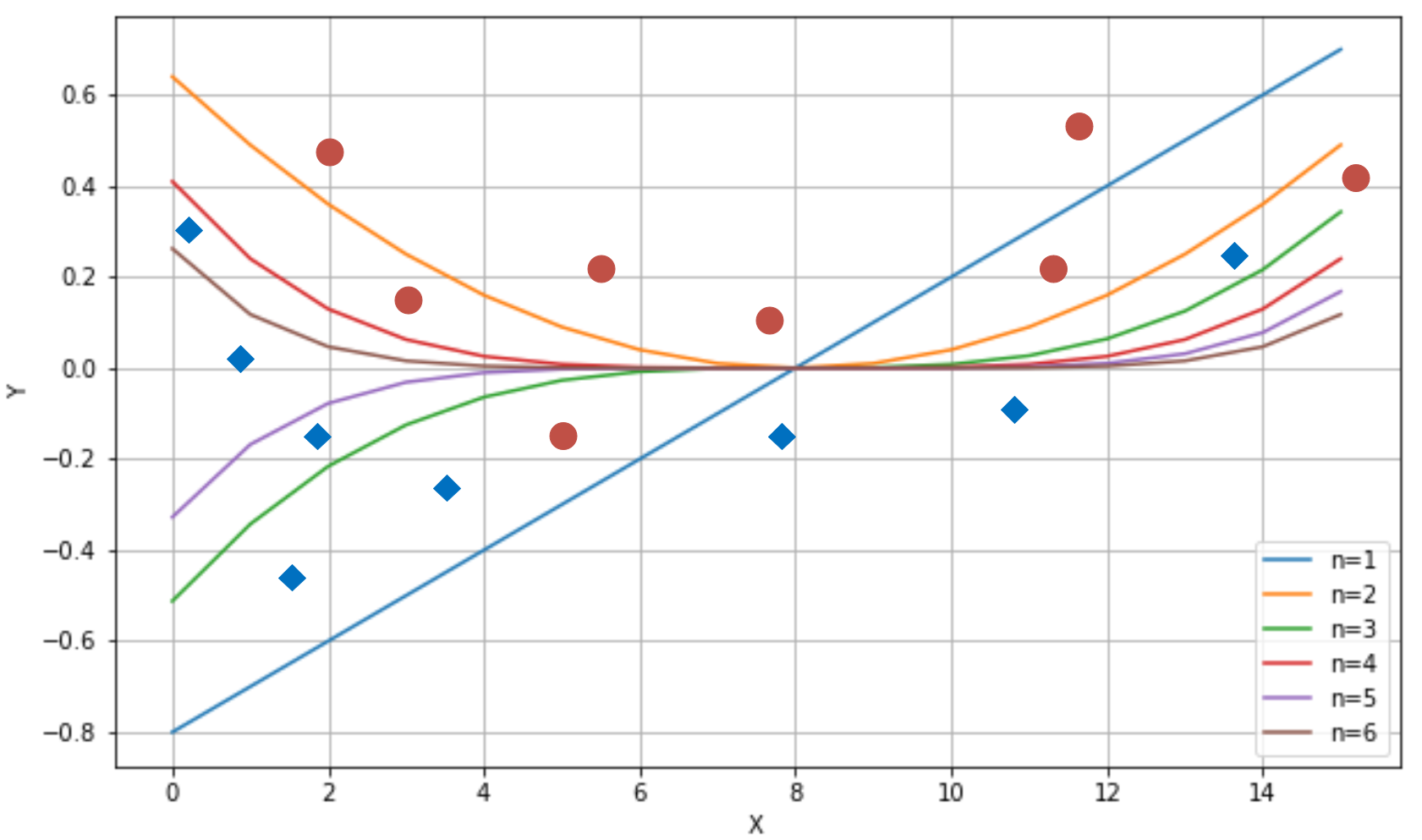Different behavior produced by six polynomial separating curves

The ability to rapidly change the curvature is proportional to the degree. If we choose a linear classifier, we can only modify its slope (the example is always in a bi-dimensional space) and the intercept. Instead, if we pick a higher-degree function, we have more possibilities to bend the curvature when it's necessary. If we consider n=1 and n=2 in the plot (on the top-right, they are the first and the second functions), with n=1, we can include the dot corresponding to x=11, but this choice has a negative impact on the dot at x=5.

Only a parameterized non-linear function can solve this problem efficiently, because this simple problem requires a representational capacity higher than the one provided by linear classifiers. Another classical example is the XOR function. For a long time, several researchers opposed perceptrons (linear neural networks), because they weren't able to classify a dataset generated by the XOR function. Fortunately, the introduction of multilayer perceptrons, with non-linear functions, allowed us to overcome this problem, and many whose complexity is beyond the possibilities of any classic machine learning model.

#### Vapnik-Chervonenkis capacity

A mathematical formalization of the capacity of a classifier is provided by the Vapnik-Chervonenkis theory. To introduce the definition, it's first necessary to define the concept of shattering. If we have a class of sets C and a set M, we say that C shatters M if:In other words, given any subset of M, it can be obtained as the intersection of a particular instance of C (cj) and M itself. If we now consider a model as a parameterized function:We want to determine its capacity in relation to a finite dataset X:According to the Vapnik-Chervonenkis theory, we can say that the model f shatters X if there are no classification errors for every possible label assignment. Therefore, we can define the Vapnik-Chervonenkis-capacity or VC-capacity (sometimes called VC-dimension) as the maximum cardinality of a subset of X so that f can shatter it.

For example, if we consider a linear classifier in a bi-dimensional space, the VC-capacity is equal to 3, because it's always possible to label three samples so that f shatters them; however, it's impossible to do it in all situations where N > 3. The XOR problem is an example that needs a VC-capacity higher than 3. Let's explore the following plot: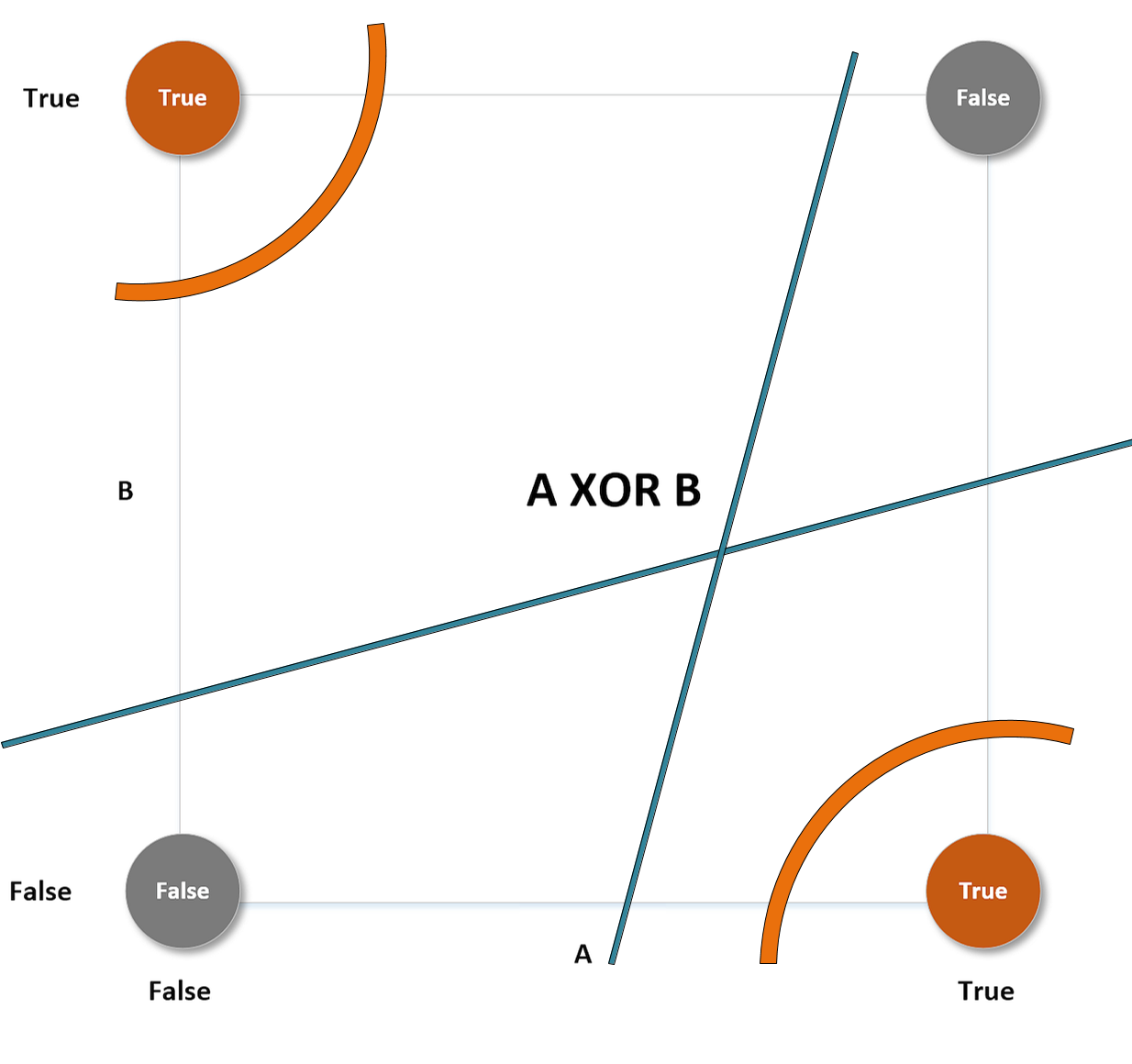XOR problem with different separating curves

This particular label choice makes the set non-linearly separable. The only way to overcome this problem is to use higher-order functions (or non-linear ones). The curve lines (belonging to a classifier whose VC-capacity is greater than 3) can separate both the upper-left and the lower-right regions from the remaining space, but no straight line can do the same (while it can always separate one point from the other three).

### Bias of an estimator

Let's now consider a parameterized model with a single vectorial parameter (this isn't a limitation, but only a didactic choice):The goal of a learning process is to estimate the parameter θ so as, for example, to maximize the accuracy of a classification. We define the bias of an estimator (in relation to a parameter θ):In other words, the bias is the difference between the expected value of the estimation and the real parameter value. Remember that the estimation is a function of X, and cannot be considered a constant in the sum.

An estimator is said to be unbiased if:Moreover, the estimator is defined as consistent if the sequence of estimations converges (at least with probability 1) to the real value when k → ∞:Given a dataset X whose samples are drawn from pdata, the accuracy of an estimator is inversely proportional to its bias. Low-bias (or unbiased) estimators are able to map the dataset X with high-precision levels, while high-bias estimators are very likely to have a capacity that isn't high enough for the problem to solve, and therefore their ability to detect the whole dynamic is poor.

Let's now compute the derivative of the bias with respect to the vector θ (it will be useful later):Consider that the last equation, thanks to the linearity of E[•], holds also if we add a term that doesn't depend on x to the estimation of θ. In fact, in line with the laws of probability, it's easy to verify that:#### Underfitting

A model with a high bias is likely to underfit the training set. Let's consider the scenario shown in the following graph: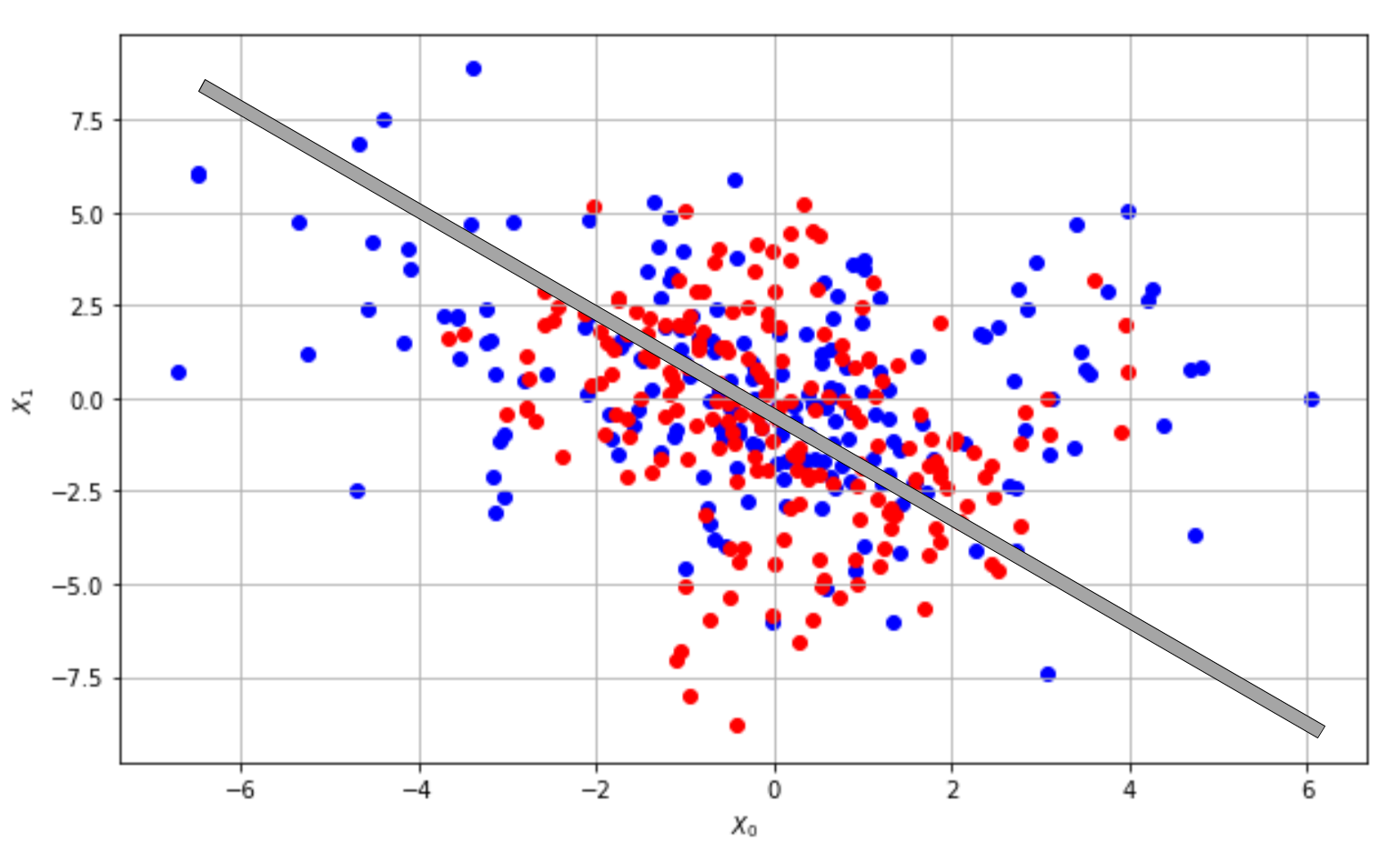Underfitted classifier: The curve cannot separate correctly the two classes

Even if the problem is very hard, we could try to adopt a linear model and, at the end of the training process, the slope and the intercept of the separating line are about -1 and 0 (as shown in the plot); however, if we measure the accuracy, we discover that it's close to 0! Independently from the number of iterations, this model will never be able to learn the association between X and Y. This condition is called underfitting, and its major indicator is a very low training accuracy. Even if some data preprocessing steps can improve the accuracy, when a model is underfitted, the only valid solution is to adopt a higher-capacity model.

In a machine learning task, our goal is to achieve the maximum accuracy, starting from the training set and then moving on to the validation set. More formally, we can say that we want to improve our models so to get as close as possible to Bayes accuracy. This is not a well-defined value, but a theoretical upper limit that is possible to achieve using an estimator. In the following diagram, we see a representation of this process: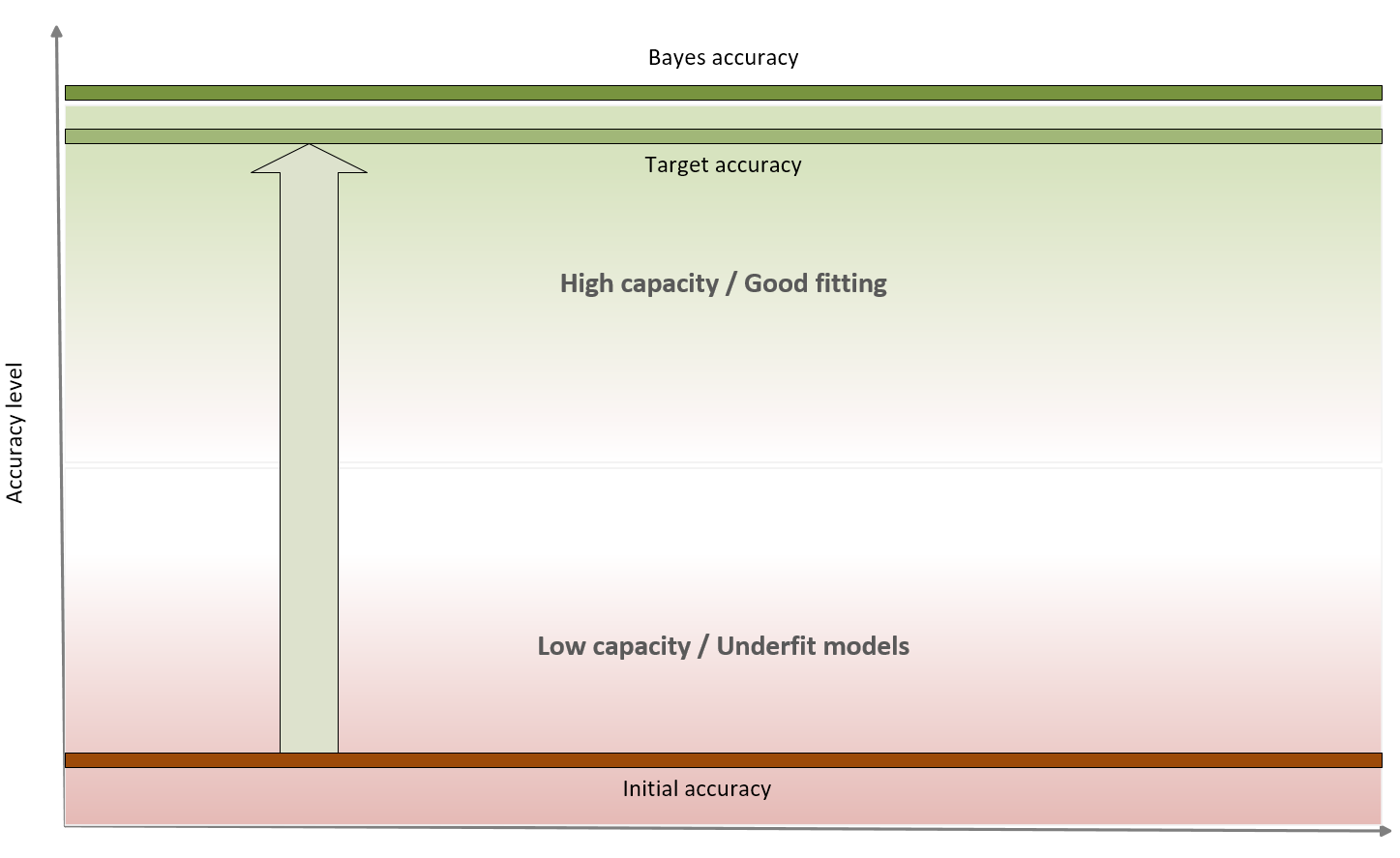Accuracy level diagram

Bayes accuracy is often a purely theoretical limit and, for many tasks, it's almost impossible to achieve using even biological systems; however, advancements in the field of deep learning allow to create models that have a target accuracy slightly below the Bayes one. In general, there's no closed form for determining the Bayes accuracy, therefore human abilities are considered as a benchmark. In the previous classification example, a human being is immediately able to distinguish among different dot classes, but the problem can be very hard for a limited-capacity classifier. Some of the models we're going to discuss can solve this problem with a very high target accuracy, but at this point, we run another risk that can be understood after defining the concept of variance of an estimator.

### Variance of an estimator

At the beginning of this chapter, we have defined the data generating process pdata, and we have assumed that our dataset X has been drawn from this distribution; however, we don't want to learn existing relationships limited to X, but we expect our model to be able to generalize correctly to any other subset drawn from pdata. A good measure of this ability is provided by the variance of the estimator:The variance can be also defined as the square of the standard error (analogously to the standard deviation). A high variance implies dramatic changes in the accuracy when new subsets are selected, because the model has probably reached a very high training accuracy through an over-learning of a limited set of relationships, and it has almost completely lost its ability to generalize.

#### Overfitting

If underfitting was the consequence of a low capacity and a high bias, overfitting is a phenomenon that a high variance can detect. In general, we can observe a very high training accuracy (even close to the Bayes level), but not a poor validation accuracy. This means that the capacity of the model is high enough or even excessive for the task (the higher the capacity, the higher the probability of large variances), and that the training set isn't a good representation of pdata. To understand the problem, consider the following classification scenarios: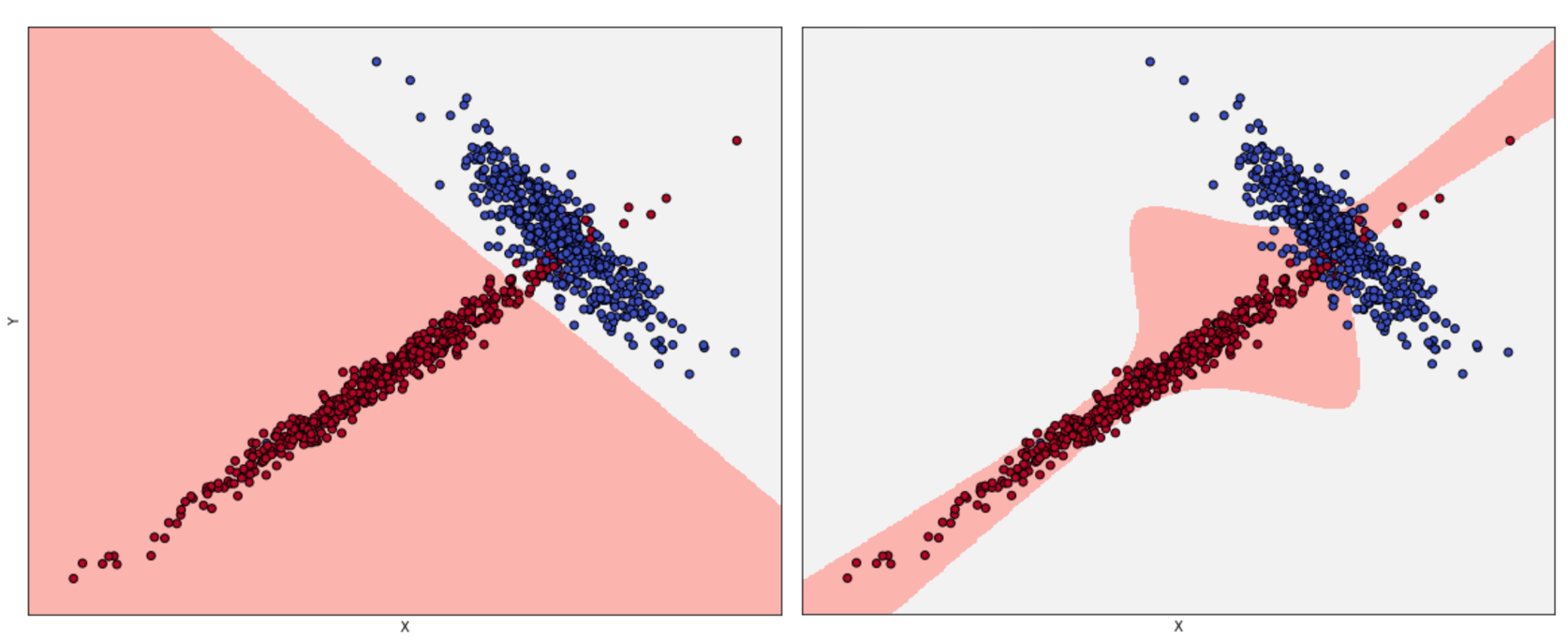Acceptable fitting (left), overfitted classifier (right)

The left plot has been obtained using logistic regression, while, for the right one, the algorithm is SVM with a sixth-degree polynomial kernel. If we consider the second model, the decision boundaries seem much more precise, with some samples just over them. Considering the shapes of the two subsets, it would be possible to say that a non-linear SVM can better capture the dynamics; however, if we sample another dataset from pdata and the diagonal tail becomes wider, logistic regression continues to classify the points correctly, while the SVM accuracy decreases dramatically. The second model is very likely to be overfitted, and some corrections are necessary. When the validation accuracy is much lower than the training one, a good strategy is to increase the number of training samples to consider the real pdata. In fact, it can happen that a training set is built starting from a hypothetical distribution that doesn't reflect the real one; or the number of samples used for the validation is too high, reducing the amount of information carried by the remaining samples. Cross-validation is a good way to assess the quality of datasets, but it can always happen that we find completely new subsets (for example, generated when the application is deployed in a production environment) that are misclassified, even if they were supposed to belong to pdata. If it's not possible to enlarge the training set, data augmentation could be a valid solution, because it allows creating artificial samples (for images, it's possible to mirror, rotate, or blur them) starting from the information stored in the known ones. Other strategies to prevent overfitting are based on a technique called regularization, which we're going to discuss in the last part of this chapter. For now, we can say that the effect of regularization is similar to a partial linearization, which implies a capacity reduction with a consequent variance decrease.

#### The Cramér-Rao bound

If it's theoretically possible to create an unbiased model (even asymptotically), this is not true for variance. To understand this concept, it's necessary to introduce an important definition: the Fisher information. If we have a parameterized model and a data-generating process pdata, we can define the likelihood function by considering the following parameters:This function allows measuring how well the model describes the original data generating process. The shape of the likelihood can vary substantially, from well-defined, peaked curves, to almost flat surfaces. Let's consider the following graph, showing two examples based on a single parameter: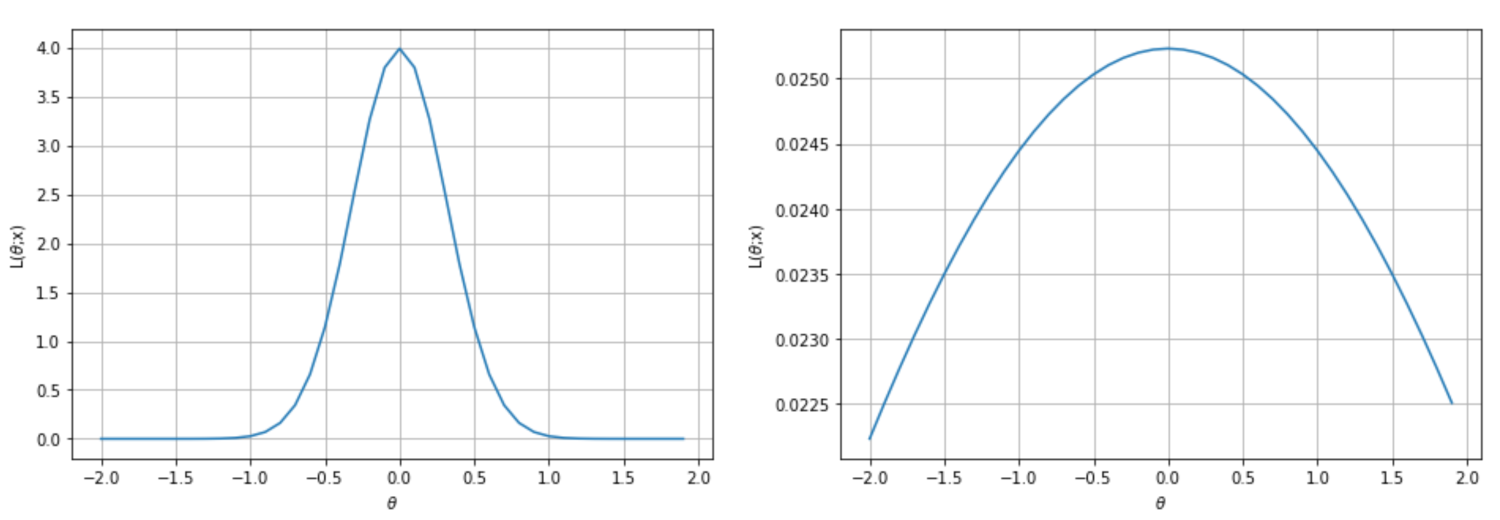Very peaked likelihood (left), flatter likelihood (right)

We can immediately understand that, in the first case, the maximum likelihood can be easily reached by gradient ascent, because the surface is very peaked. In the second case, instead, the gradient magnitude is smaller, and it's rather easy to stop before reaching the actual maximum because of numerical imprecisions or tolerances. In worst cases, the surface can be almost flat in very large regions, with a corresponding gradient close to zero. Of course, we'd like to always work with very sharp and peaked likelihood functions, because they carry more information about their maximum. More formally, the Fisher information quantifies this value. For a single parameter, it is defined as follows:The Fisher information is an unbounded non-negative number that is proportional to the amount of information carried by the log-likelihood; the use of logarithm has no impact on the gradient ascent, but it simplifies complex expressions by turning products into sums. This value can be interpreted as the speed of the gradient when the function is reaching the maximum; therefore, higher values imply better approximations, while a hypothetical value of zero means that the probability to determine the right parameter estimation is also null.

When working with a set of K parameters, the Fisher information becomes a positive semidefinite matrix:This matrix is symmetric, and also has another important property: when a value is zero, it means that the corresponding couple of parameters are orthogonal for the purpose of the maximum likelihood estimation, and they can be considered separately. In many real cases, if a value is close to zero, it determines a very low correlation between parameters and, even if it's not mathematically rigorous, it's possible to decouple them anyway.

At this point, it's possible to introduce the Cramér-Rao bound, which states that for every unbiased estimator that adopts x (with probability distribution p(x; θ)) as a measure set, the variance of any estimator of θ is always lower-bounded according to the following inequality:In fact, considering initially a generic estimator and exploiting Cauchy-Schwarz inequality with the variance and the Fisher information (which are both expressed as expected values), we obtain:Now, if we use the expression for derivatives of the bias with respect to θ, considering that the expected value of the estimation of θ doesn't depend on x, we can rewrite the right side of the inequality as:If the estimator is unbiased, the derivative on the right side is equal to zero, therefore, we get:In other words, we can try to reduce the variance, but it will be always lower-bounded by the inverse Fisher information. Therefore, given a dataset and a model, there's always a limit to the ability to generalize. In some cases, this measure is easy to determine; however, its real value is theoretical, because it provides the likelihood function with another fundamental property: it carries all the information needed to estimate the worst case for variance. This is not surprising: when we discussed the capacity of a model, we saw how different functions could drive to higher or lower accuracies. If the training accuracy is high enough, this means that the capacity is appropriate or even excessive for the problem; however, we haven't considered the role of the likelihood p(X| θ).

High-capacity models, in particular, with small or low-informative datasets, can drive to flat likelihood surfaces with a higher probability than lower-capacity models. Therefore, the Fisher information tends to become smaller, because there are more and more parameter sets that yield similar probabilities, and this, at the end of the day, drives to higher variances and an increased risk of overfitting. To conclude this section, it's useful to consider a general empirical rule derived from the Occam's razor principle: whenever a simpler model can explain a phenomenon with enough accuracy, it doesn't make sense to increase its capacity. A simpler model is always preferable (when the performance is good and it represents accurately the specific problem), because it's normally faster both in the training and in the inference phases, and more efficient. When talking about deep neural networks, this principle can be applied in a more precise way, because it's easier to increase or decrease the number of layers and neurons until the desired accuracy has been achieved.

## Loss and cost functions

At the beginning of this chapter, we discussed the concept of generic target function so as to optimize in order to solve a machine learning problem. More formally, in a supervised scenario, where we have finite datasets X and Y:We can define the generic loss function for a single sample as:J is a function of the whole parameter set, and must be proportional to the error between the true label and the predicted. Another important property is convexity. In many real cases, this is an almost impossible condition; however, it's always useful to look for convex loss functions, because they can be easily optimized through the gradient descent method. We're going to discuss this topic in Chapter 9, Neural Networks for Machine Learning. However, for now, it's useful to consider a loss function as an intermediate between our training process and a pure mathematical optimization. The missing link is the complete data. As already discussed, X is drawn from pdata, so it should represent the true distribution. Therefore, when minimizing the loss function, we're considering a potential subset of points, and never the whole real dataset. In many cases, this isn't a limitation, because, if the bias is null and the variance is small enough, the resulting model will show a good generalization ability (high training and validation accuracy); however, considering the data generating process, it's useful to introduce another measure called expected risk:This value can be interpreted as an average of the loss function over all possible samples drawn from pdata. Minimizing the expected risk implies the maximization of the global accuracy. When working with a finite number of training samples, instead, it's common to define a cost function (often called a loss function as well, and not to be confused with the log-likelihood):This is the actual function that we're going to minimize and, divided by the number of samples (a factor that doesn't have any impact), it's also called empirical risk, because it's an approximation (based on real data) of the expected risk. In other words, we want to find a set of parameters so that:When the cost function has more than two parameters, it's very difficult and perhaps even impossible to understand its internal structure; however, we can analyze some potential conditions using a bidimensional diagram: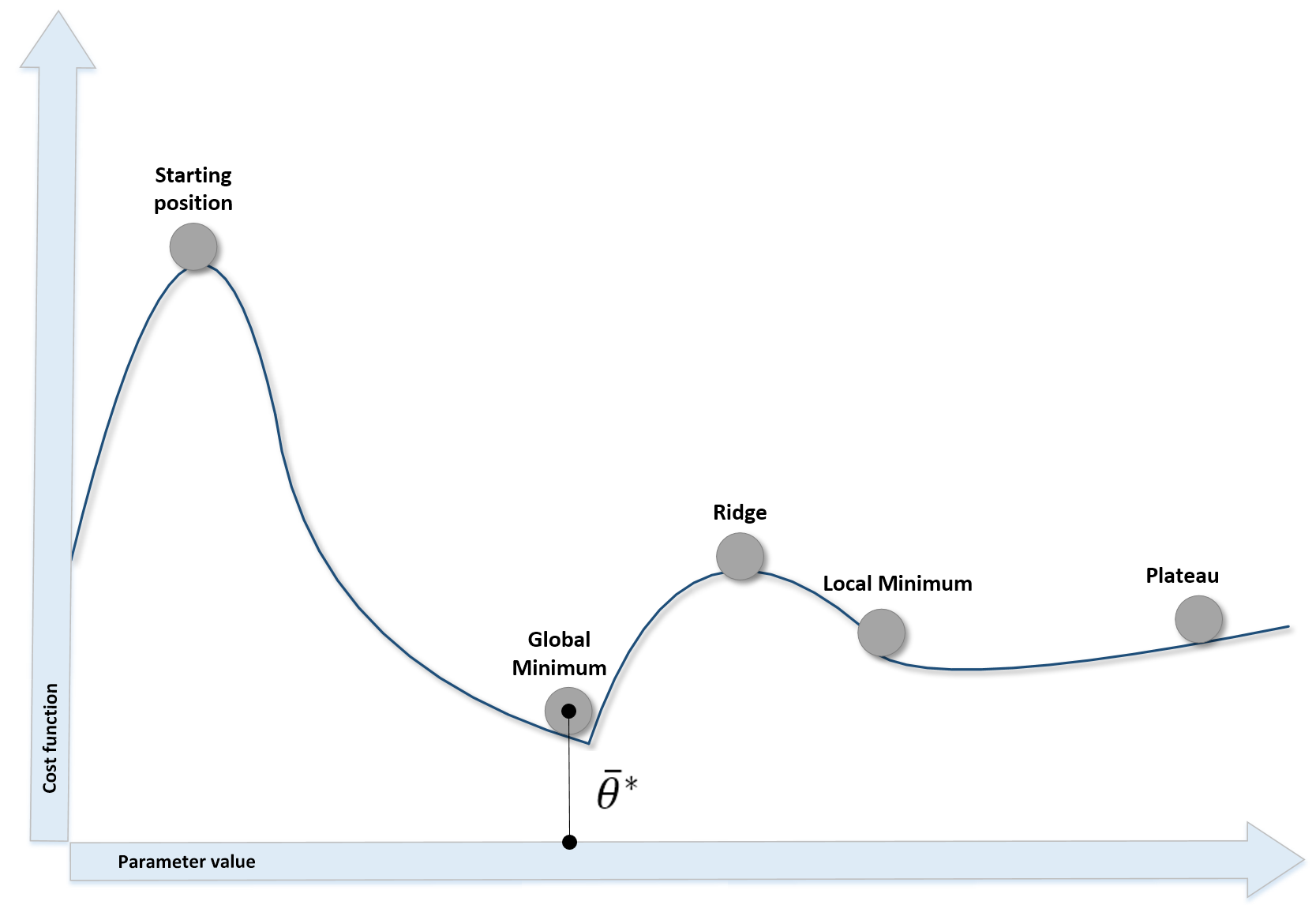Different kinds of points in a bidimensional scenario

The different situations we can observe are:

• The starting point, where the cost function is usually very high due to the error.
• Local minima, where the gradient is null (and the second derivative is positive). They are candidates for the optimal parameter set, but unfortunately, if the concavity isn't too deep, an inertial movement or some noise can easily move the point away.
• Ridges (or local maxima), where the gradient is null, and the second derivative is negative. They are unstable points, because a minimum perturbation allows escaping, reaching lower-cost areas.
• Plateaus, or the region where the surface is almost flat and the gradient is close to zero. The only way to escape a plateau is to keep a residual kinetic energy—we're going to discuss this concept when talking about neural optimization algorithms (Chapter 9, Neural Networks for Machine Learning).
• Global minimum, the point we want to reach to optimize the cost function.

Even if local minima are likely when the number of parameters is small, they become very unlikely when the model has a large number of parameters. In fact, an n-dimensional point θ* is a local minimum for a convex function (and here, we're assuming L to be convex) only if:The second condition imposes a positive semi-definite Hessian matrix (equivalently, all principal minors Hn made with the first n rows and n columns must be non-negative), therefore all its eigenvalues λ0, λ1, ..., λN must be non-negative. This probability decreases with the number of parameters (H is a n×n square matrix and has n eigenvalues), and becomes close to zero in deep learning models where the number of weights can be in the order of 10,000,000 (or even more). The reader interested in a complete mathematical proof can read High Dimensional Spaces, Deep Learning and Adversarial Examples, Dube S., arXiv:1801.00634 [cs.CV]. As a consequence, a more common condition to consider is instead the presence of saddle points, where the eigenvalues have different signs and the orthogonal directional derivatives are null, even if the points are neither local maxima nor minima. Consider, for example, the following plot: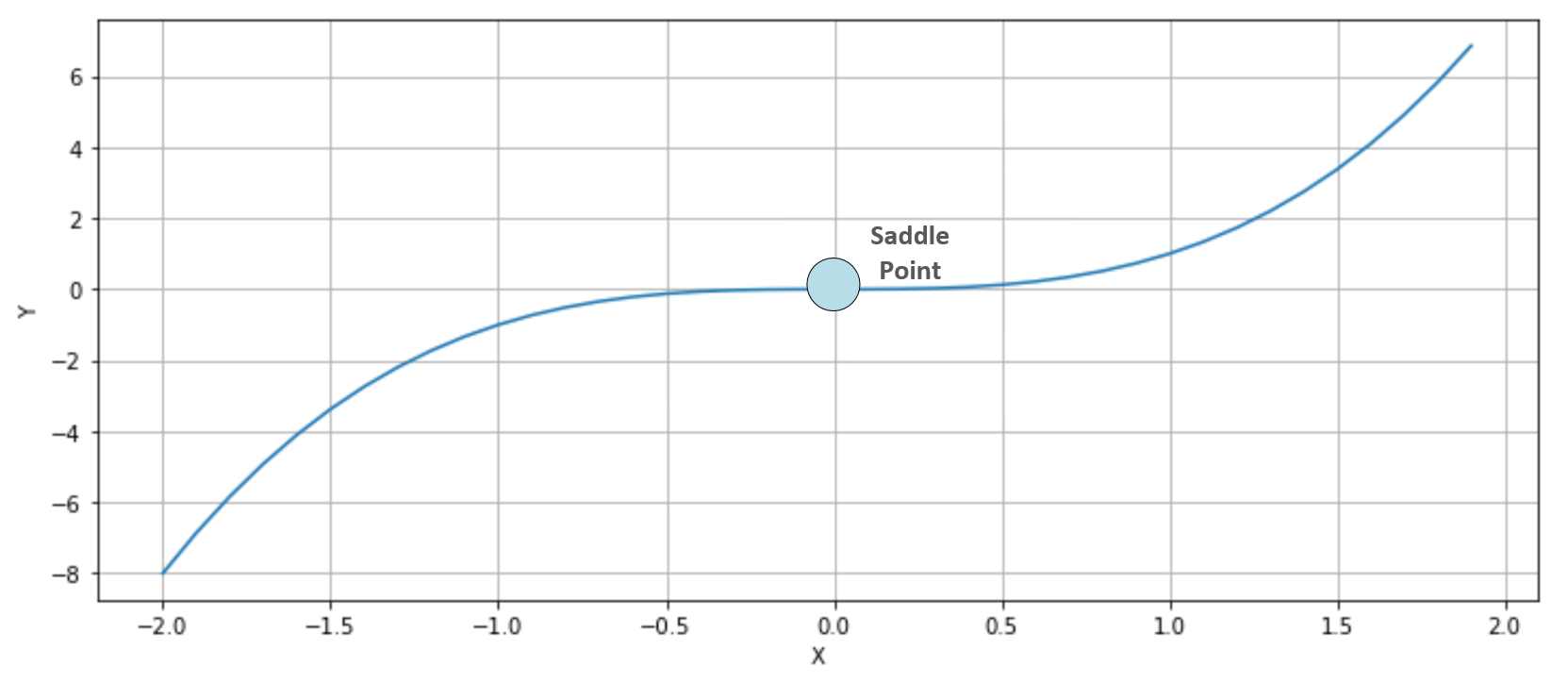Saddle point in a bidimensional scenario

The function is y=x3 whose first and second derivatives are y'=3x2 and y''=6x. Therefore, y'(0)=y''(0)=0. In this case (single-valued function), this point is also called a point of inflection, because at x=0, the function shows a change in the concavity. In three dimensions, it's easier to understand why a saddle point has been called in this way. Consider, for example, the following plot: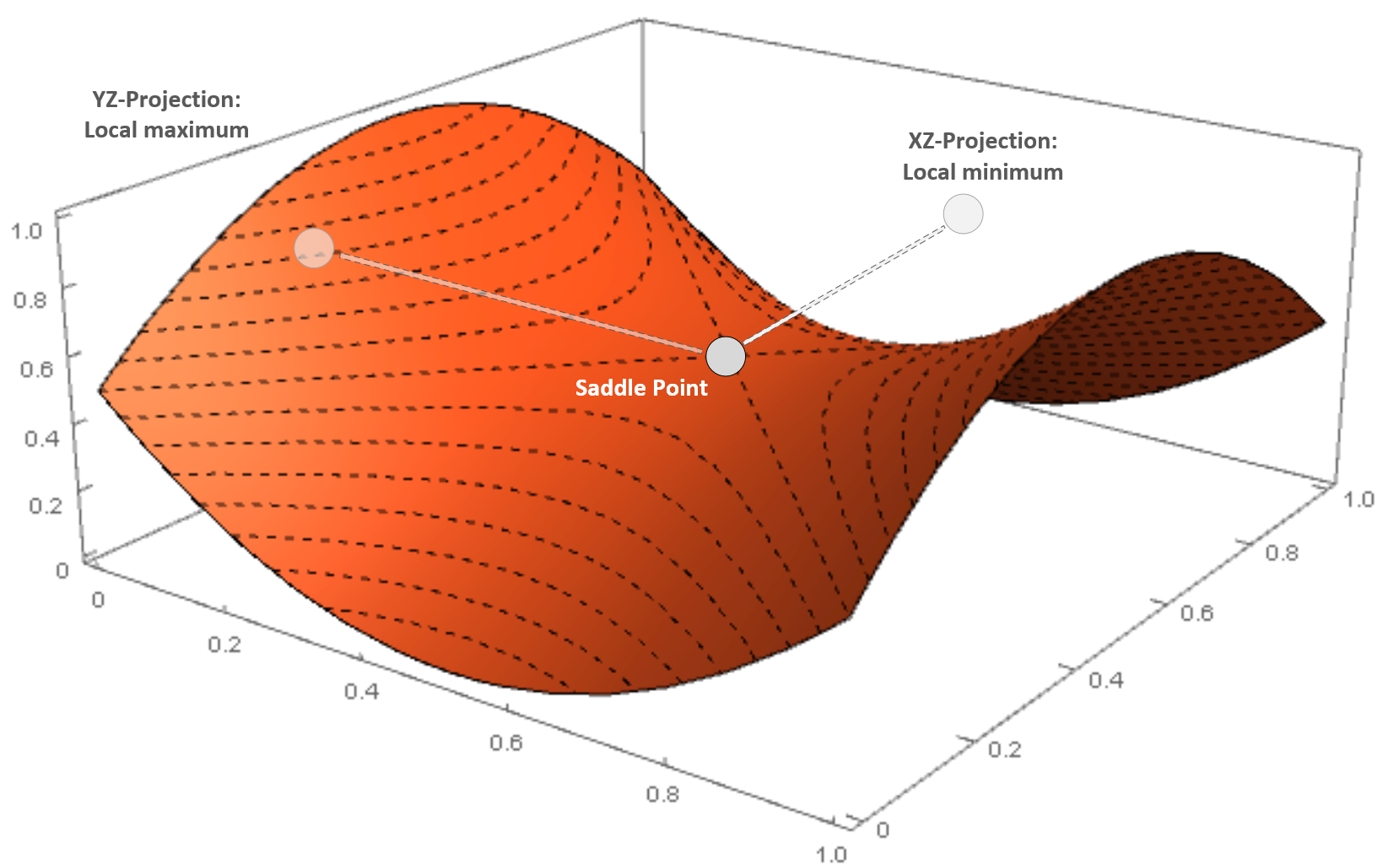Saddle point in a three-dimensional scenario

The surface is very similar to a horse saddle, and if we project the point on an orthogonal plane, XZ is a minimum, while on another plane (YZ) it is a maximum. Saddle points are quite dangerous, because many simpler optimization algorithms can slow down and even stop, losing the ability to find the right direction. In Chapter 9, Neural Networks for Machine Learning, we're going to discuss some methods that are able to mitigate this kind of problem, allowing deep models to converge.

### Examples of cost functions

In this section, we expose some common cost functions that are employed in both classification and regression tasks. Some of them will be extensively adopted in our examples in the next chapters, particularly when discussing training processes in shallow and deep neural networks.

#### Mean squared error

Mean squared error is one of the most common regression cost functions. Its generic expression is:This function is differentiable at every point of its domain and it's convex, so it can be optimized using the stochastic gradient descent (SGD) algorithm; however, there's a drawback when employed in regressions where there are outliers. As its value is always quadratic when the distance between the prediction and the actual value (corresponding to an outlier) is large, the relative error is high, and this can lead to an unacceptable correction.

#### Huber cost function

As explained, mean squared error isn't robust to outliers, because it's always quadratic independently of the distance between actual value and prediction. To overcome this problem, it's possible to employ the Hubercost function, which is based on threshold tH, so that for distances less than tH, its behavior is quadratic, while for a distance greater than tH, it becomes linear, reducing the entity of the error and, therefore, the relative importance of the outliers.

The analytical expression is:#### Hinge cost function

This cost function is adopted by SVM, where the goal is to maximize the distance between the separation boundaries (where the support vector lies). It's analytic expression is:Contrary to the other examples, this cost function is not optimized using classic stochastic gradient descent methods, because it's not differentiable at all points where:For this reason, SVM algorithms are optimized using quadratic programming techniques.

#### Categorical cross-entropy

Categorical cross-entropy is the most diffused classification cost function, adopted by logistic regression and the majority of neural architectures. The generic analytical expression is:This cost function is convex and can be easily optimized using stochastic gradient descent techniques; moreover, it has another important interpretation. If we are training a classifier, our goal is to create a model whose distribution is as similar as possible to pdata. This condition can be achieved by minimizing the Kullback-Leibler divergence between the two distributions:In the previous expression, pM is the distribution generated by the model. Now, if we rewrite the divergence, we get:The first term is the entropy of the data-generating distribution, and it doesn't depend on the model parameters, while the second one is the cross-entropy. Therefore, if we minimize the cross-entropy, we also minimize the Kullback-Leibler divergence, forcing the model to reproduce a distribution that is very similar to pdata. This is a very elegant explanation as to why the cross-entropy cost function is an excellent choice for classification problems.

### Regularization

When a model is ill-conditioned or prone to overfitting, regularization offers some valid tools to mitigate the problems. From a mathematical viewpoint, a regularizer is a penalty added to the cost function, so to impose an extra-condition on the evolution of the parameters:The parameter λ controls the strength of the regularization, which is expressed through the function g(θ). A fundamental condition on g(θ) is that it must be differentiable so that the new composite cost function can still be optimized using SGD algorithms. In general, any regular function can be employed; however, we normally need a function that can contrast the indefinite growth of the parameters.

To understand the principle, let's consider the following diagram: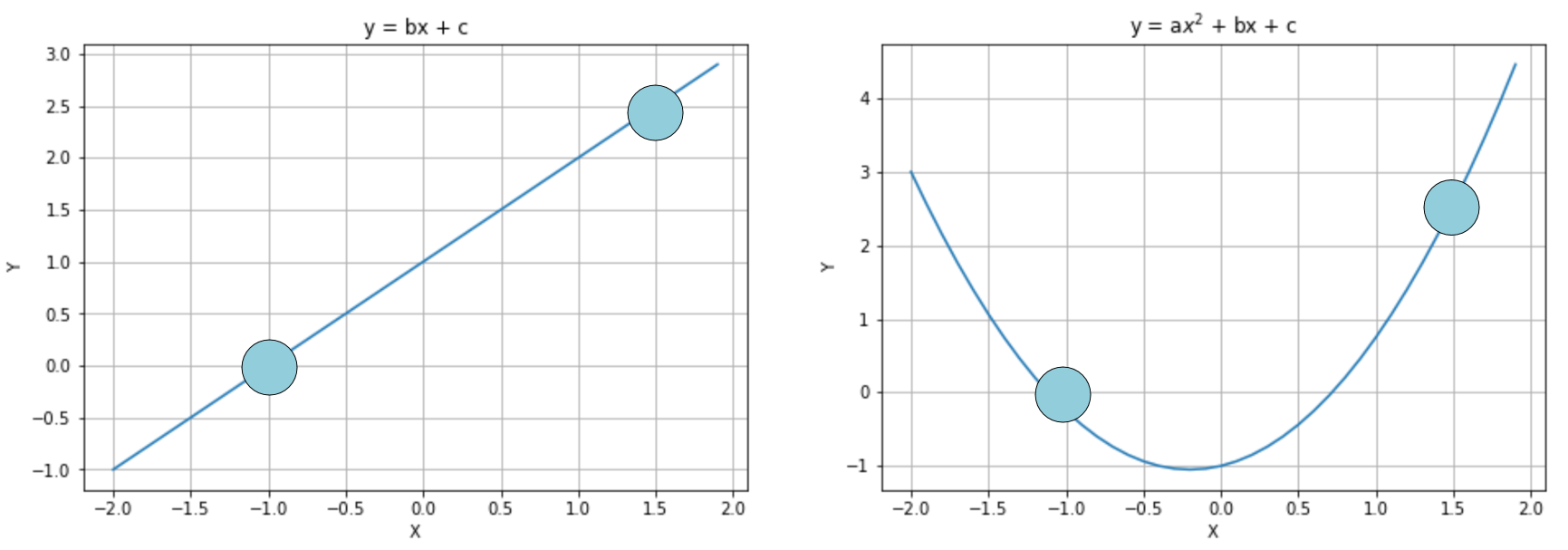Interpolation with a linear curve (left) and a parabolic one (right)

In the first diagram, the model is linear and has two parameters, while in the second one, it is quadratic and has three parameters. We already know that the second option is more prone to overfitting, but if we apply a regularization term, it's possible to avoid the growth of a (first quadratic parameter), transforming the model into a linearized version. Of course, there's a difference between choosing a lower-capacity model and applying a regularization constraint. In fact, in the first case, we are renouncing the possibility offered by the extra capacity, running the risk of increasing the bias, while with regularization we keep the same model but optimize it so to reduce the variance. Let's now explore the most common regularization techniques.

#### Ridge

Ridge regularization (also known as Tikhonov regularization) is based on the squared L2-norm of the parameter vector:This penalty avoids an infinite growth of the parameters (for this reason, it's also known as weight shrinkage), and it's particularly useful when the model is ill-conditioned, or there is multicollinearity, due to the fact that the samples are completely independent (a relatively common condition).

In the following diagram, we see a schematic representation of the Ridge regularization in a bidimensional scenario: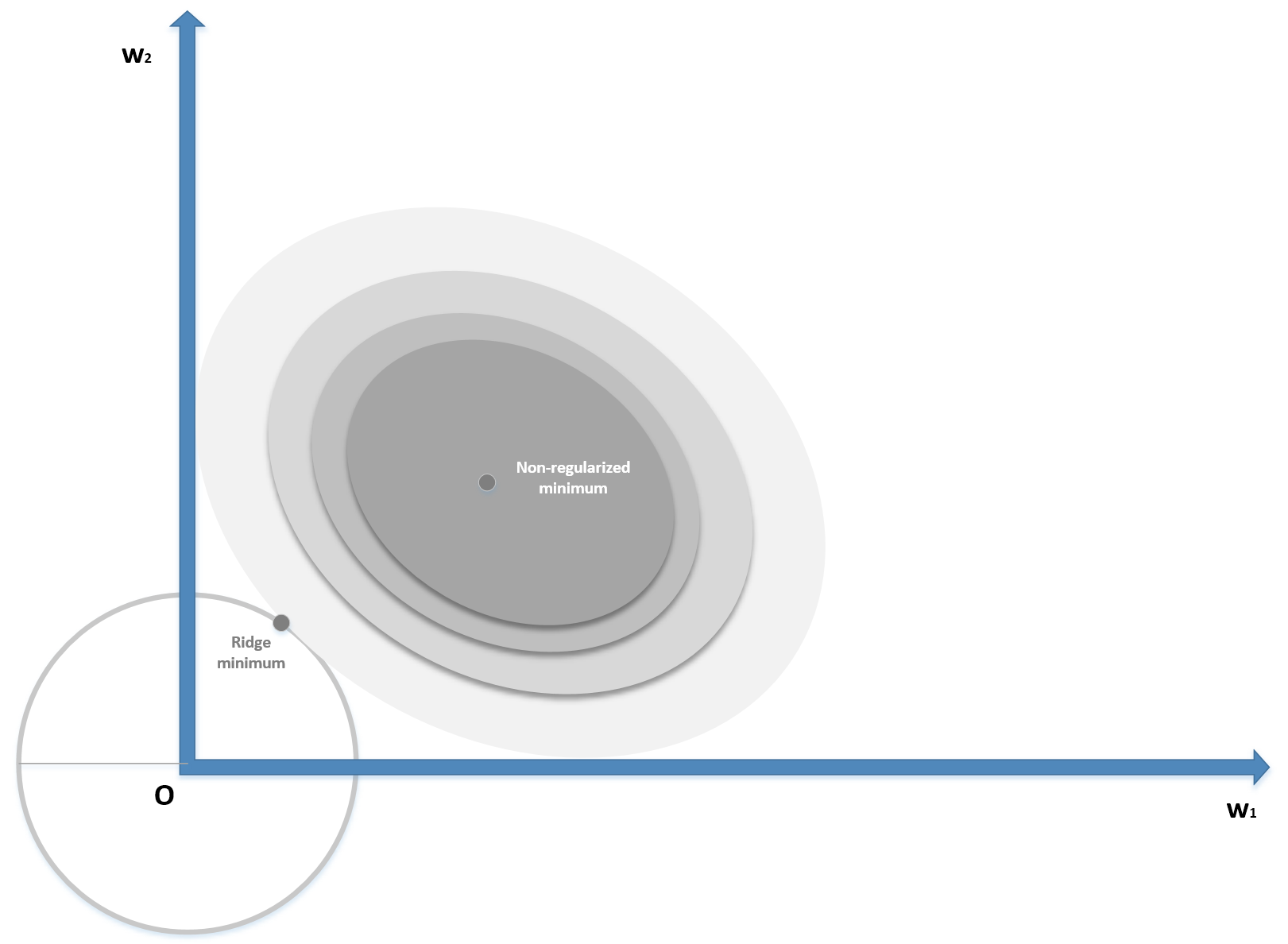Ridge (L2) regularization

The zero-centered circle represents the Ridge boundary, while the shaded surface is the original cost function. Without regularization, the minimum (w1, w2) has a magnitude (for example, the distance from the origin) which is about double the one obtained by applying a Ridge constraint, confirming the expected shrinkage. When applied to regressions solved with the Ordinary Least Squares (OLS) algorithm, it's possible to prove that there always exists a Ridge coefficient, so that the weights are shrunk with respect the OLS ones. The same result, with some restrictions, can be extended to other cost functions.

#### Lasso

Lasso regularization is based on the L1-norm of the parameter vector:Contrary to Ridge, which shrinks all the weights, Lasso can shift the smallest one to zero, creating a sparse parameter vector. The mathematical proof is beyond the scope of this book; however, it's possible to understand it intuitively by considering the following diagram (bidimensional):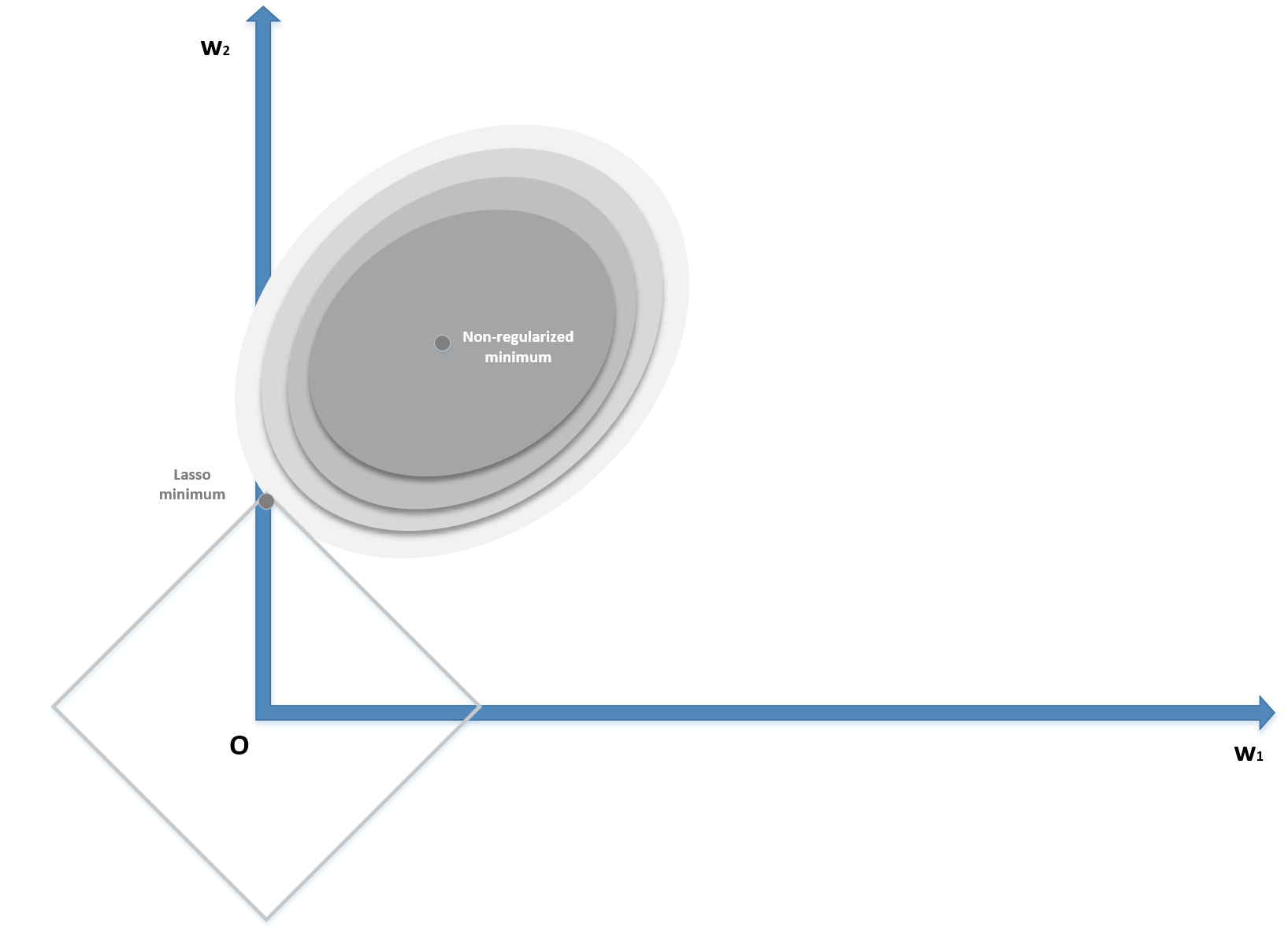Lasso (L1) regularization

The zero-centered square represents the Lasso boundaries. If we consider a generic line, the probability of being tangential to the square is higher at the corners, where at least one (exactly one in a bidimensional scenario) parameter is null. In general, if we have a vectorial convex function f(x) (we provide a definition of convexity in Chapter 5, EM Algorithm and Applications), we can define:As any Lp-norm is convex, as well as the sum of convex functions, g(x) is also convex. The regularization term is always non-negative, therefore the minimum corresponds to the norm of the null vector. When minimizing g(x), we need to also consider the contribution of the gradient of the norm in the ball centered in the origin where, however, the partial derivatives don't exist. Increasing the value of p, the norm becomes smoothed around the origin, and the partial derivatives approach zero for |xi| → 0.

On the other side, with p=1 (excluding the L0-norm and all the norms with p ∈ ]0, 1[ that allow an even stronger sparsity, but are non-convex), the partial derivatives are always +1 or -1, according to the sign of xi (xi ≠ 0). Therefore, it's easier for the L1-norm to push the smallest components to zero, because the contribution to the minimization (for example, with a gradient descent) is independent of xiwhile an L2-norm decreases its speed when approaching the origin. This is a non-rigorous explanation of the sparsity achieved using the L1-norm. In fact, we also need to consider the term f(x), which bounds the value of the global minimum; however, it may help the reader to develop an intuitive understanding of the concept. It's possible to find further and mathematically rigorous details in Optimization for Machine Learning, (edited by) Sra S., Nowozin S., Wright S. J., The MIT Press.

Lasso regularization is particularly useful whenever a sparse representation of a dataset is needed. For example, we could be interested in finding the feature vectors corresponding to a group of images. As we expect to have many features but only a subset present in each image, applying the Lasso regularization allows forcing all the smallest coefficients to become null, suppressing the presence of the secondary features. Another potential application is latent semantic analysis, where our goal is to describe the documents belonging to a corpus in terms of a limited number of topics. All these methods can be summarized in a technique called sparse coding, where the objective is to reduce the dimensionality of a dataset (also in non-linear scenarios) by extracting the most representative atoms, using different approaches to achieve sparsity.

#### ElasticNet

In many real cases, it's useful to apply both Ridge and Lasso regularization in order to force weight shrinkage and a global sparsity. It is possible by employing the ElasticNet regularization, defined as:The strength of each regularization is controlled by the parameters λ1 and λ2. ElasticNet can yield excellent results whenever it's necessary to mitigate overfitting effects while encouraging sparsity. We are going to apply all the regularization techniques when discussing some deep learning architectures.

#### Early stopping

Even though it's a pure regularization technique, early stopping is often considered as a last resort when all other approaches to prevent overfitting and maximize validation accuracy fail. In many cases (above all, in deep learning scenarios), it's possible to observe a typical behavior of the training process considering both training and the validation cost functions: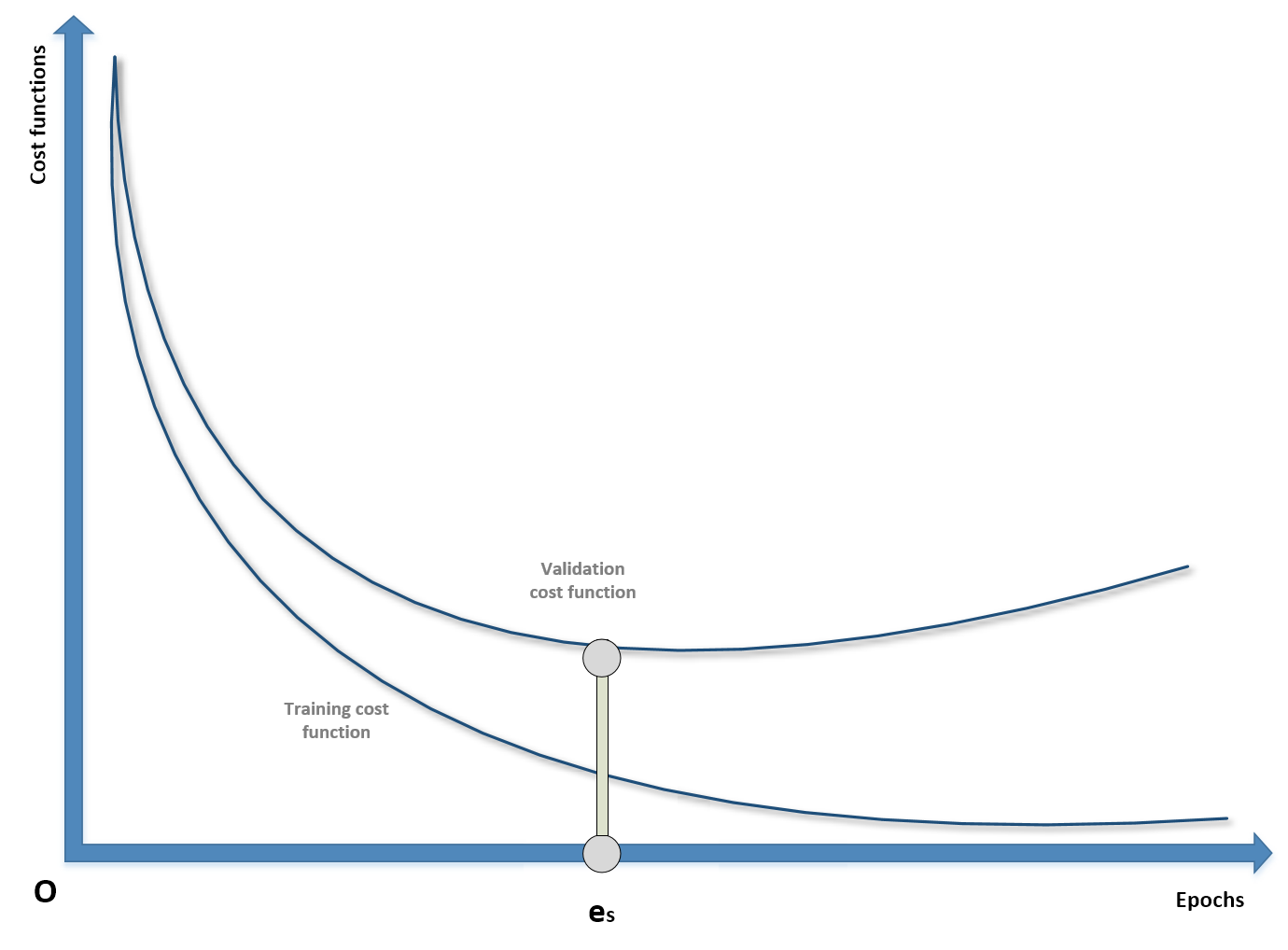Example of early stopping before the beginning of ascending phase of U-curve

During the first epochs, both costs decrease, but it can happen that after a threshold epoch es, the validation cost starts increasing. If we continue with the training process, this results in overfitting the training set and increasing the variance. For this reason, when there are no other options, it's possible to prematurely stop the training process. In order to do so, it's necessary to store the last parameter vector before the beginning of a new iteration and, in the case of no improvements or the accuracy worsening, to stop the process and recover the last parameters. As explained, this procedure must never be considered as the best choice, because a better model or an improved dataset could yield higher performances. With early stopping, there's no way to verify alternatives, therefore it must be adopted only at the last stage of the process and never at the beginning. Many deep learning frameworks such as Keras include helpers to implement an early stopping callback; however, it's important to check whether the last parameter vector is the one stored before the last epoch or the one corresponding to es. In this case, it could be useful to repeat the training process, stopping it at the epoch previous to es (where the minimum validation cost has been achieved).

## Summary

In this chapter, we discussed fundamental concepts shared by almost any machine learning model. In the first part, we have introduced the data generating process, as a generalization of a finite dataset. We explained which are the most common strategies to split a finite dataset into a training block and a validation set, and we introduced cross-validation, with some of the most important variants, as one of the best approaches to avoid the limitations of a static split.

In the second part, we discussed the main properties of an estimator: capacity, bias, and variance. We also introduced the Vapnik-Chervonenkis theory, which is a mathematical formalization of the concept of representational capacity, and we analyzed the effects of high biases and high variances. In particular, we discussed effects called underfitting and overfitting, defining the relationship with high bias and high variance.

In the third part, we introduced the loss and cost functions, first as proxies of the expected risk, and then we detailed some common situations that can be experienced during an optimization problem. We also exposed some common cost functions, together with their main features. In the last part, we discussed regularization, explaining how it can mitigate the effects of overfitting.

In the next chapter, Chapter 2Introduction to Semi-Supervised Learning, we're going to introduce semi-supervised learning, focusing our attention on the concepts of transductive and inductive learning.